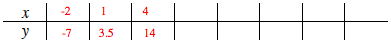### Home > MC1 > Chapter 9 > Lesson 9.2.5 > Problem9-109

9-109.

Find at least six (x, y) pairs for the rule $\frac { y } { x } = \frac { 7 } { 2 }$ and organize them into a table like the one below. Be sure to use positive and negative numbers.

Can you find fractions that are equivalent to seven-halves? You can use the Giant One or any other method you like.

$\text{It is also likely that multiples of 7 and 2 will produce an ordered pair following the rule }\frac{7}{2}.$

Just be sure that if you pick one multiple, the other multiple corresponds.
For instance, 7 times 5 is 35, so to form an ordered pair we need to find the fifth multiple of 2.Some possible pairs are listed in the table above.
Can you find more pairs to complete the table?
Remember to use negative numbers too!